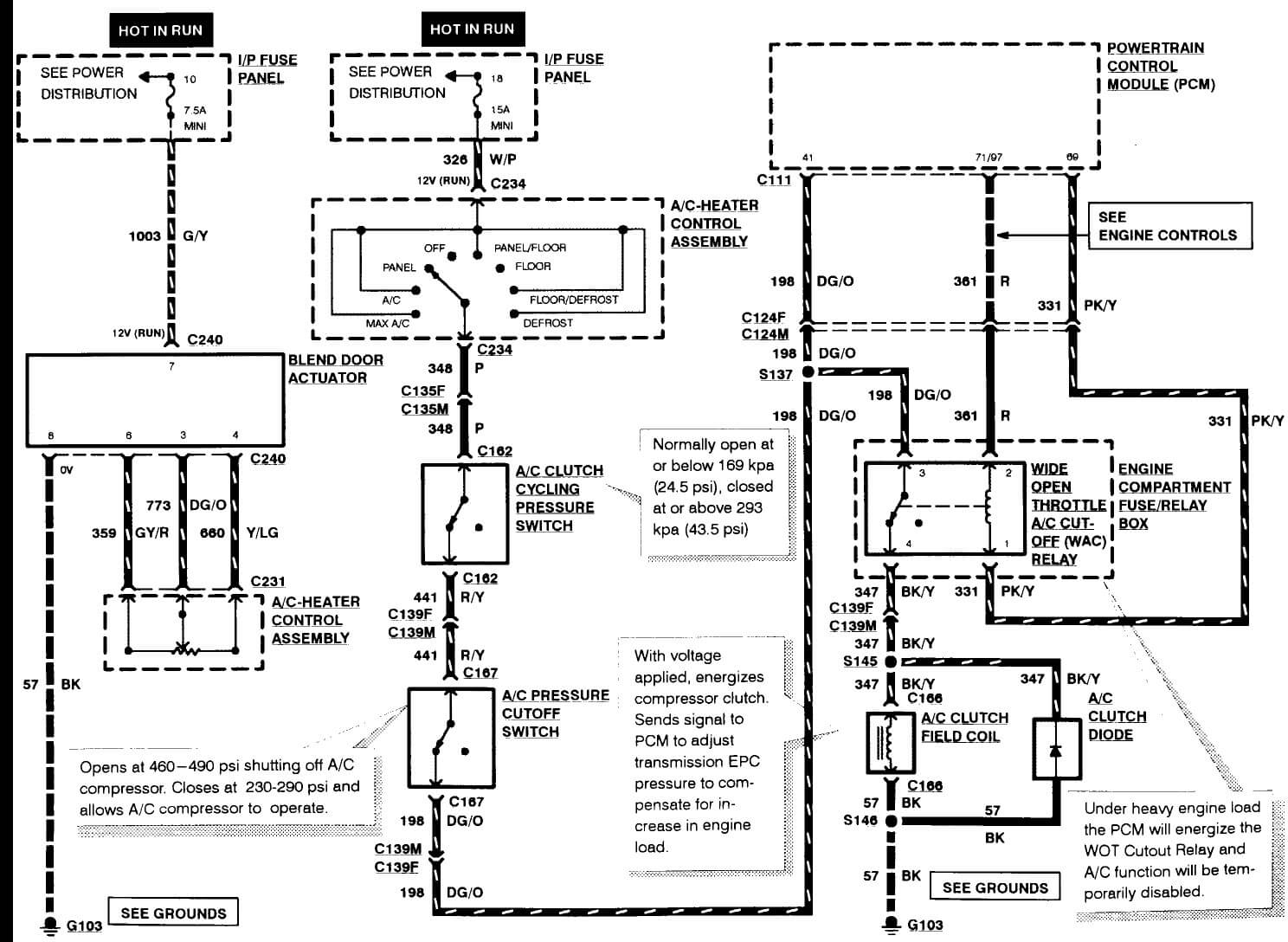UPDATED 2021

# Free Test For Testing

### Numbers and Operations

 1. Which answer is a temperature?½x² ¼x³ ¾π 25°F 2--32 14÷2
 2. True or false: 8 ≥ 5 true false neither both
This is the second scenario
 3. Question 6 1 2 3 4
 4. Question 5 a b c d e
 5. Question 4 1 2 3 4
This is the first scenario
 6. Question 3 1 2 3 4
 7. Question 2 1 2 3 4
 8. Question 1 A B C D
 9. 4 + 2 = _ 2 4 6 8
 10. 4 - 2 = _ 2 4 6 8
 11. 4 × 2 = _ 2 4 6 8
 12. 4 ÷ 2 = _ 2 4 6 8
 13. The area of a circle can be calculated by A = πr A = 2πr A = πr² A = πD